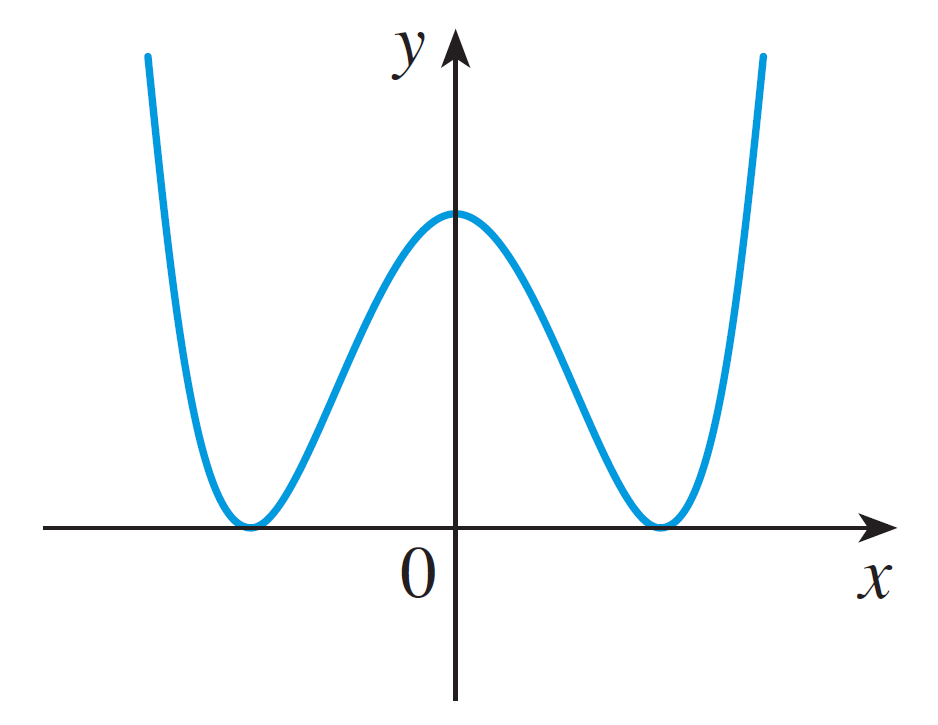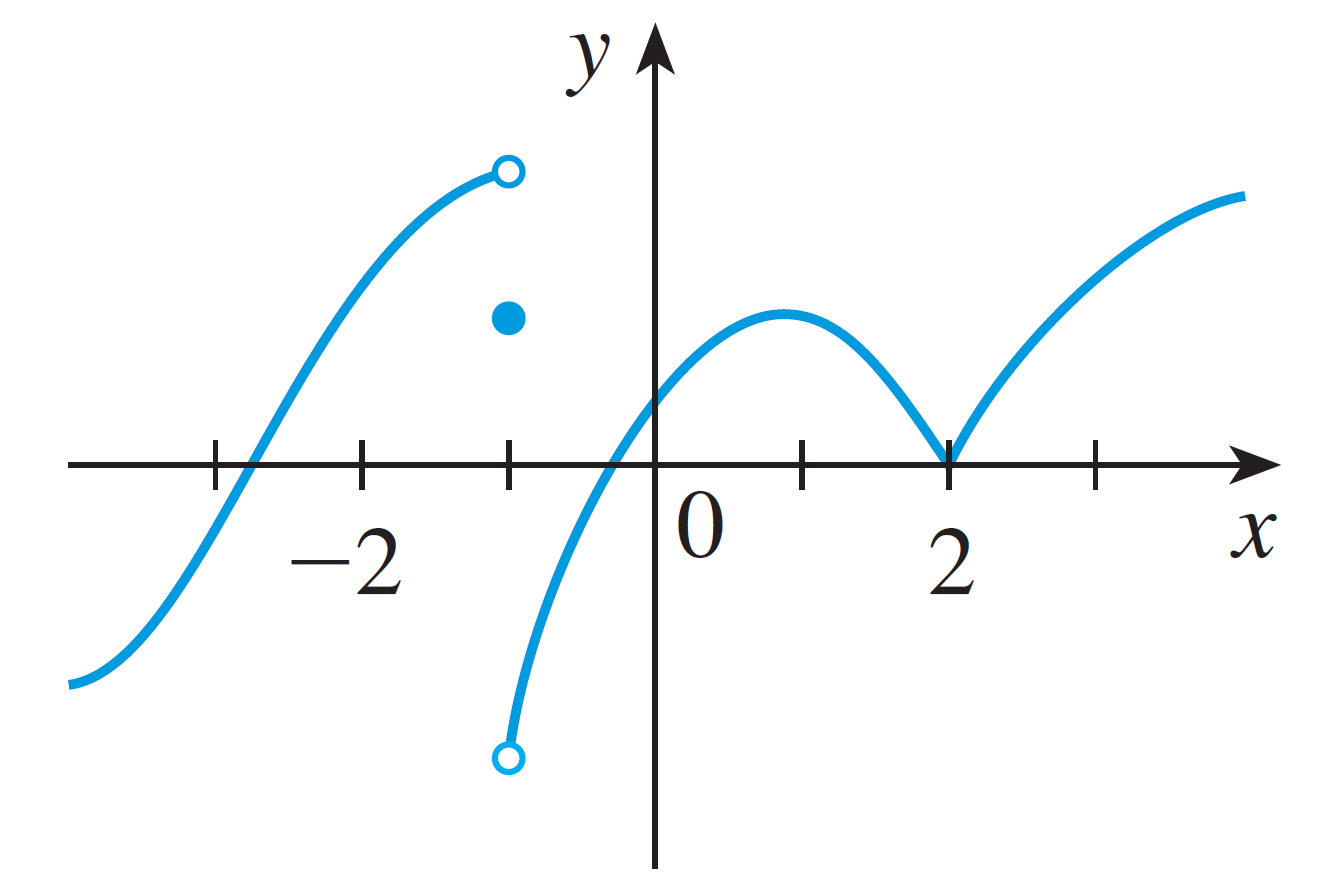# Homework 5

Directions:

1. Show each step of your work and fully simplify each expression.
2. Turn in your answers in class on a physical piece of paper.
3. Staple multiple sheets together.
4. Feel free to use Desmos for graphing.

1. Show $f(x) = x^4 + x - 3$ has a root between $(1, 2)$.
2. Explain why $f(x) = \dfrac{\sin x}{x + 1}$ is continuous on $(\infty, -1) \cup (-1, \infty)$.
3. When we are using the definition of the derivative:
1. What always happens to the $h$ in the denominator?
2. Suppose the above phenomena does not happen. What is the problem?
4. Suppose $f(x)$ is differentiable on $\mathbb{R}$. Must $f(x)$ be continuous on $\mathbb{R}$? If not, explain why.
5. Suppose $f(x)$ is continuous on $\mathbb{R}$. Must $f(x)$ be differentiable on $\mathbb{R}$? If not, draw the graph of a function where it fails to be differentiable.
6. Find an equation of the tangent line at $(2, -4)$ for the function $f(x) = 4x - 3x^2$.
7. Using the definition of a derivative, find $f'(x)$.
1. $f(x) = 2x + 3$
2. $f(x) = x + \sqrt{x}$
3. $f(x) = x^2 - 1$
4. $f(t) = \dfrac{1}{\sqrt{t}}$
8. Suppose the graph of $f(x)$ isSketch a graph of $f'(x)$.
9. The graph of $f$ is given. State with reason where $f$ is not differentiable. You may assume the domain of $f$ is $\mathbb{R}$.10. Find the derivative.
1. $f(x) = 199$
2. $g(t) = \left(\sqrt{30}\right)^3$
3. $f(x) = x^3 + x^2 + x + 1$
4. $f(v) = \dfrac{a}{v} + bv + \dfrac{c}{v^2}$
5. $f(x) = \sqrt{x}(x - 1)$
6. $f(t) = 3t^{-3/4}$
7. $f(x) = (-3)^2$
8. $f(x) = x^{2.9}$
9. $f(r) = -9r^{2/3}$
10. $f(x) = 5x^2 + 6x + 7$
11. $f(r) = \dfrac{r^5 + 2r^4 + 3r + 1}{r}$
12. $f(a) = \dfrac{5}{a^4} - \dfrac{2}{\sqrt{a^2}} -\dfrac{1}{a} + 200$
13. $f(x) = \dfrac{5}{x^4} + \dfrac{\sqrt{x^3}}{3} + x$
14. $f(x) = 2x\left(3x^2 + 1\right)$